• 均方误差(Mean Square Error, MSE)损失函数： 对求偏导（对求导类似）： 交叉熵损失函数： 对求偏导（对求导类似）： ...MSE损失函数在参数更新的过程会依赖激活函数的偏导数，当激...
均方误差(Mean Square Error, MSE)损失函数：

对求偏导（对求导类似）：

交叉熵损失函数：

对求偏导（对求导类似）：

ps：以上公式中，激活函数取sigmod激活函数

参数更新过程：

对比公式(2)和(4)可知：

MSE损失函数在参数更新的过程会依赖激活函数的偏导数，当激活函数取sigmod函数的时候，其偏导数在自变量很大或很小的时候为0，使得训练过程中网络参数无更新。

而交叉熵损失函数在参数更新的过程中不依赖激活函数的偏导数，不存在MSE的问题。

展开全文• pytorch求梯度， MSE损失函数用sum和mean的区别 1. z.mean()取均值操作，梯度计算如下 import torch x = torch.ones(2, 2, requires_grad=True) print(x) # tensor([[1., 1.], # [1., 1.]], requires_grad=True) ...
pytorch求梯度， MSE损失函数用sum和mean的区别

1.  z.mean()取均值操作，梯度计算如下

import torch
print(x)
# tensor([[1., 1.],

y = x + 2
print(y)
# tensor([[3., 3.],

z = y * y * 3
print(z)
# tensor([[27., 27.],

out = z.mean()
print(out)

out.backward() # 梯度回传，必须要这一步才能计算z对x的梯度
# tensor([[4.5000, 4.5000],
#        [4.5000, 4.5000]])



解析：
z=3*y*y=3*(x+2)(x+2)
out=z.mean=
out 对各个x_i 求导
将x的值带入，得到结果
# tensor([[4.5000, 4.5000],
#        [4.5000, 4.5000]])

2.  z.sum()取求和操作，梯度计算如下

import torch
print(x1)
# tensor([[1., 1.],

y1 = x1 + 2
print(y1)
# tensor([[3., 3.],

z1 = y1 * y1 * 3
print(z1)
# tensor([[27., 27.],

out2 = z1.sum()
print(out2)

out2.backward() # 梯度回传，必须要这一步才能计算z对x的梯度
# tensor([[18., 18.],
#        [18., 18.]])


解析：
z1=3*y1*y1=3*(x1+2)(x1+2)
out2=z1.sum=
out2 对x1 求导 =
将x的值带入，得到结果
# tensor([[18., 18.],
#        [18., 18.]])

展开全文python 深度学习
• 1.1激活函数 激活函数：引入非线性激活因素，提高模型的表达能力 常用的激活函数有relu、sigmoid、tanh等 (1)激活函数relu：在Tensorflow中，用tf.nn.relu()表示 (2)激活函数sigmoid：在Tensorflow中，用tf.nn....
一. 定义

1.1激活函数

激活函数：引入非线性激活因素，提高模型的表达能力
常用的激活函数有relu、sigmoid、tanh等
(1)激活函数relu：在Tensorflow中，用tf.nn.relu()表示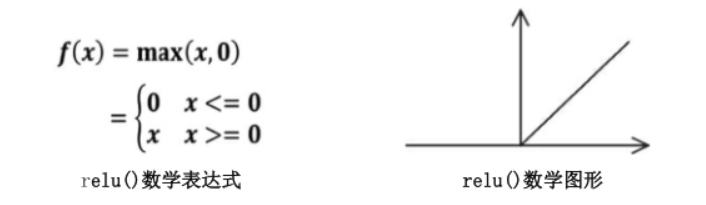(2)激活函数sigmoid：在Tensorflow中，用tf.nn.sigmoid()表示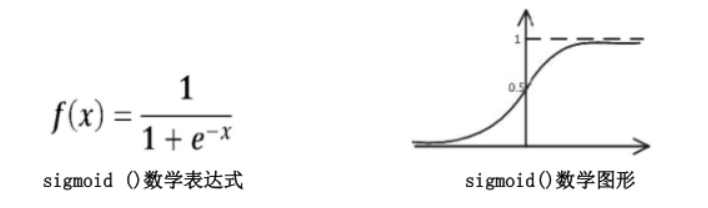(3)激活函数tanh：在Tensorflow中，用tf.nn.tanh()表示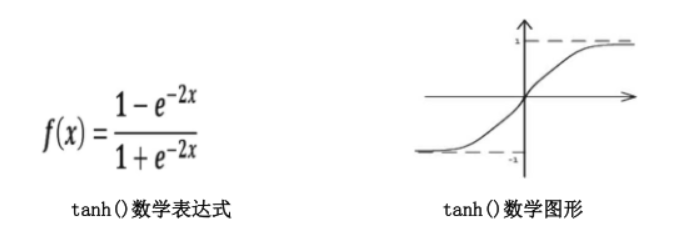1.2神经元

神经元模型：用数学公式比表示为：f(Σi xi*wi + b), f为激活函数
神经网络 是以神经元为基本单位构成的
神经网络的复杂度：可用神经网络的的层数和神经网络中待优化参数个数表示
神经网络的层数：一般不计入输入层，层数 = n个隐藏层 + 1个输出层
神经网络待优化的参数：神经网络中所有参数w的个数 + 所有参数b的个数在该神经网络中，包含1个输入层，1个隐藏层和1个输出层，该神经网络的参数为2层
在该神经网络中，参数的个数是所有参数w的个数加上所有参数b的总数，第一层参数用三行四列的二阶张量表示（即12个线上的权重w）再加上4个偏置b；第二层参数是四行二列的二阶张量（即8个线上的权重w）再加上2个偏置b
总参数 = 34+4 + 42+2 = 26
二. 损失函数

损失函数（loss）：用来表示预测（y）与已知答案（y_）的差距。在训练神经网络时，通过不断改变神经网络中所有参数，使损失函数不断减小，从而训练出更高准确率的神经网络模型
常用的损失函数有均方误差，自定义和交叉熵等
2.1 MSE均方误差

均方误差mse：n个样本的预测值（y）与（y_）的差距。在训练神经网络时，通过不断的改变神经网络中的所有参数，使损失函数不断减小，从而训练出更高准确率的神经网络模型。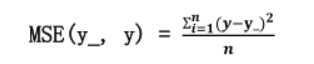在Tensorflow中用loss_mse = tf.reduce_mean(tf.square(y_-y))
具体实现：

预测酸奶日销量 y，x1 和 x2 是影响日销量的两个因素。应提前采集的数据有：一段时间内，每日的 x1 因素、x2 因素和销量 y_。采集的数据尽量多。在本例中用销量预测产量，最优的产量应该等于销量。由于目前没有数据集，所以拟造了一套数
据集。利用 Tensorflow 中函数随机生成 x1、 x2，制造标准答案 y_ = = x1 + x2，为了更真实，求和后还加了正负 0.05 的随机噪声。我们把这套自制的数据集喂入神经网络，构建一个一层的神经网络，拟合预测酸奶日销量的函数。

代码实现：

#预测酸奶日销量 y，x1 和 x2 是影响日销量的两个因素。
#应提前采集的数据有：一段时间内，每日的 x1 因素、x2 因素和销量 y_。采集的数据尽量多。
#在本例中用销量预测产量，最优的产量应该等于销量。由于目前没有数据集，所以拟造了一套数
#据集。利用 函数随机生成 x1、 x2，制造标准答案 y_ = = x1 + x2，为了更真实，求和后
#还加了正负 0.05 的随机噪声。
#我们把这套自制的数据集喂入神经网络，构建一个一层的神经网络，拟合预测酸奶日销量的函数
import tensorflow as tf
import numpy as np
BATCH_SIZE = 8
SEED = 23455

#基于seed产生随机数
rdm = np.random.RandomState(SEED)
#随机数返回32行2列的矩阵  表示32组   体积和重量   作为输入数据集
X = rdm.rand(32, 2)
#作为输入数据集的标签（正确答案）
Y_ = [[x1+x2+(rdm.rand()/10.0-0.05)] for(x1, x2) in X]

#定义神经网络的输入、参数和输出，定义前向传播过程
x = tf.placeholder(tf.float32, shape=(None, 2))
w1 = tf.Variable(tf.random_normal([2, 1], stddev=1, seed=1))
y_ = tf.placeholder(tf.float32, shape=(None, 1))    #正确答案，标签
y = tf.matmul(x, w1)    #N*1，预测答案

#定义损失函数以及反向传播方法
#损失函数为MSE，反向传播方法为梯度下降
loss_mse = tf.reduce_mean(tf.square(y_ - y))

#生成会话session，训练STEP轮
with tf.Session() as sess:
#初始化参数
init_op = tf.global_variables_initializer()
sess.run(init_op)

#训练模型，最关键步骤
STEPS = 20000   #迭代次数
for i in range(STEPS):
start = (i*BATCH_SIZE) % 32
end = start + BATCH_SIZE
sess.run(train_step, feed_dict={x: X[start:end], y_: Y_[start:end]})     #喂数据，不断更新参数
if i % 500 == 0:    #每迭代500次输出一次结果  损失函数loss在所有训练集上的损失值
#total_loss = sess.run(loss, feed_dict={x: X, y_: Y_})
#print("After %d training step(s), loss on all data is %g" % (i, total_loss))
print("After %d training steps, w1 is: " % i)
print(sess.run(w1))
print("\n")
print("Final w1 is: ")  #打印最终参数w1
print(sess.run(w1))
#最终输出参数为0.98和1.02，销量预测结果为 y=0.98x1 + 1.02x2，标准答案为 y=x1 + x2
# 销量预测结果和标准答案已非常接近，说明该神经网络预测酸奶日销量正确

实现结果（部分截取）：

#随着训练次数的增加，线上的参数不断变化
After 19000 training steps, w1 is:
[[0.974931 ]
[1.0206276]]

After 19500 training steps, w1 is:
[[0.9777026]
[1.0181949]]

Final w1 is:
[[0.98019385]
[1.0159807 ]]

结果分析：

有上述代码可知，本例中神经网络预测模型为y = w1x1 + w2x2，损失函数采用均方误差。通过使损失函数值（loss）不断降低，神经网络模型得到最终参数w1 = 0.98，w2 = 1.02，销量预测结果为y = 0.98x1 + 1.02x2。由于在生成数据集时，标准答案为y = x1 + x2，因此，销量预测结果和标准答案已经非常接近，说明该神经网络预测酸奶日销量正确。

2.2自定义损失函数

自定义损失函数：根据问题的实际情况，定制合理的损失函数
具体例子分析：
对于预测酸奶日销量问题，如果预测销量大于实际销量则会损失成本；如果预测销量小于实际销量则会损失利润。在实际生活中，往往制造一盒酸奶的成本和销售一盒酸奶的利润不是等价的。因此，需要使用符合该问题的自定义损失函数
自定义损失函数为：loss = Σnf(y_, y)
其中，损失函数成分段函数：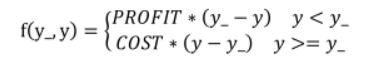Tensorflow函数表示为：loss = tf.reduce_sum(tf.where(tf.greater(y, y_), COST(y-y_), PROFIT(y_-y)))

损失函数表示：
若预测结果y小于标准答案y_，损失函数为利润乘以预测结果y与标准答案之差
若预测结果y大于标准答案y_，损失函数为成本乘以预测结果y与标准答案之差

具体例子分析：

（1）第1种情况：若酸奶成本COST为1元，酸奶销售利润PROFIT为9元，则制造成本小于酸奶利润。COST数值比PROFIT小，预测多的情况下比预测少的情况下，损失值更小 。定义损失函数使得预测多的损失小，于是模型应该偏向多的方向预测。

实现代码：

#对于预测酸奶日销量问题，如果预测销量大于实际销量则会损失成本cost；如果预测销量小于实际销量则会损失利润profit
#若预测结果 y 小于标准答案 y_，损失函数为利润profit乘以预测结果 y 与标准答案 y_之差；
#若预测结果 y 大于标准答案 y_，损失函数为成本cost乘以预测结果 y 与标准答案 y_之差
import tensorflow as tf
import numpy as np
BATCH_SIZE = 8
SEED = 23455
COST = 1    #成本
PROFIT = 9  #利润

#基于seed产生随机数
rdm = np.random.RandomState(SEED)
#随机数返回32行2列的矩阵  表示32组   体积和重量   作为输入数据集
X = rdm.rand(32, 2)
#作为输入数据集的标签（正确答案）
Y_ = [[x1+x2+(rdm.rand()/10.0-0.05)] for(x1, x2) in X]

#定义神经网络的输入、参数和输出，定义前向传播过程
x = tf.placeholder(tf.float32, shape=(None, 2))
w1 = tf.Variable(tf.random_normal([2, 1], stddev=1, seed=1))
y_ = tf.placeholder(tf.float32, shape=(None, 1))    #正确答案，标签
y = tf.matmul(x, w1)    #N*1，预测答案

#定义损失函数（自定义）以及反向传播方法
#COST数值比PROFIT小，预测多的情况下比预测少的情况下，损失值更小
#定义损失函数使得预测多的损失小，于是模型应该偏向多的方向预测
loss = tf.reduce_sum(tf.where(tf.greater(y, y_), (y - y_)*COST, (y_ - y)*PROFIT))

#生成会话session，训练STEP轮
with tf.Session() as sess:
#初始化参数
init_op = tf.global_variables_initializer()
sess.run(init_op)

#训练模型，最关键步骤
STEPS = 3000   #迭代次数
for i in range(STEPS):
start = (i*BATCH_SIZE) % 32
end = start + BATCH_SIZE
sess.run(train_step, feed_dict={x: X[start:end], y_: Y_[start:end]})     #喂数据，不断更新参数
if i % 500 == 0:    #每迭代500次输出一次结果  损失函数loss在所有训练集上的损失值
#total_loss = sess.run(loss, feed_dict={x: X, y_: Y_})
#print("After %d training step(s), loss on all data is %g" % (i, total_loss))
print("After %d training steps, w1 is: " % i)
print(sess.run(w1))
print("\n")
print("Final w1 is: ")  #打印最终参数w1
print(sess.run(w1))
#神经网络最终参数为 w1=1.03， w2=1.05，销量预测结果为 y =1.03*x1 + 1.05*x2
# 由此可见，采用自定义损失函数预测的结果大于采用均方误差预测的结果，更符合实际需求

实现结果（部分截取）：

After 2000 training steps, w1 is:
[[1.0179386]
[1.041272 ]]

After 2500 training steps, w1 is:
[[1.0205938]
[1.0390443]]

Final w1 is:
[[1.0296593]
[1.0484141]]

结果分析：

由代码执行结果可知，神经网络最终参数为w1=1.03，w2=1.05，销量预测结果为y = 1.03x1 + 1.05x2。由此可见，采用自定义损失函数预测的结果大于采用均方误差的结果，更符合实际需求。

（2）第2种情况：若酸奶成本为9元，酸奶销售利润为1元，则制造利润小于酸奶成本，COST数值比PROFIT大，预测多的情况下比预测少的情况下，损失值更大 ，定义损失函数使得预测多的损失大，于是模型应该偏向少的方向预测。

实现代码：

#对于预测酸奶日销量问题，如果预测销量大于实际销量则会损失成本cost；如果预测销量小于实际销量则会损失利润profit
#若预测结果 y 小于标准答案 y_，损失函数为利润profit乘以预测结果 y 与标准答案 y_之差；
#若预测结果 y 大于标准答案 y_，损失函数为成本cost乘以预测结果 y 与标准答案 y_之差
import tensorflow as tf
import numpy as np
BATCH_SIZE = 8
SEED = 23455
COST = 9
PROFIT = 1

#基于seed产生随机数
rdm = np.random.RandomState(SEED)
#随机数返回32行2列的矩阵  表示32组   体积和重量   作为输入数据集
X = rdm.rand(32, 2)
#作为输入数据集的标签（正确答案）
Y_ = [[x1+x2+(rdm.rand()/10.0-0.05)] for(x1, x2) in X]

#定义神经网络的输入、参数和输出，定义前向传播过程
x = tf.placeholder(tf.float32, shape=(None, 2))
w1 = tf.Variable(tf.random_normal([2, 1], stddev=1, seed=1))
y_ = tf.placeholder(tf.float32, shape=(None, 1))    #正确答案，标签
y = tf.matmul(x, w1)    #N*1，预测答案

#定义损失函数（自定义）以及反向传播方法
#COST数值比PROFIT大，预测多的情况下比预测少的情况下，损失值更大
#定义损失函数使得预测多的损失大，于是模型应该偏向少的方向预测
loss = tf.reduce_sum(tf.where(tf.greater(y, y_), (y - y_)*COST, (y_ - y)*PROFIT))

#生成会话session，训练STEP轮
with tf.Session() as sess:
#初始化参数
init_op = tf.global_variables_initializer()
sess.run(init_op)

#训练模型，最关键步骤
STEPS = 3000   #迭代次数
for i in range(STEPS):
start = (i*BATCH_SIZE) % 32
end = start + BATCH_SIZE
sess.run(train_step, feed_dict={x: X[start:end], y_: Y_[start:end]})     #喂数据，不断更新参数
if i % 500 == 0:    #每迭代500次输出一次结果  损失函数loss在所有训练集上的损失值
#total_loss = sess.run(loss, feed_dict={x: X, y_: Y_})
#print("After %d training step(s), loss on all data is %g" % (i, total_loss))
print("After %d training steps, w1 is: " % i)
print(sess.run(w1))
print("\n")
print("Final w1 is: ")  #打印最终参数w1
print(sess.run(w1))
#经网络最终参数为 w1=0.96，w2=0.97，销量预测结果为 y =0.96*x1 + 0.97*x2
#采用自定义损失函数预测的结果小于采用均方误差预测的结果，更符合实际需求

实现结果（部分截取）：

After 2000 training steps, w1 is:
[[0.9602475]
[0.9742084]]

After 2500 training steps, w1 is:
[[0.96100295]
[0.96993417]]

Final w1 is:
[[0.9600407 ]
[0.97334176]]

结果分析：

由执行结果可知，神经网络最终参数为w1 = 0.96，w2 = 0.97，销量预测结果为y = 0.96+x1 + 0.7*x2。
因此，采用自定义损失函数预测的结果小于采用均方误差预测得结果，更符合实际需求

2.3 交叉熵损失函数

交叉熵（Cross Entropy）：表示两个概率分布之间的距离，交叉熵越大，两个概率分布距离越远，两个概率分布越相异；交叉熵越小，两个概率分布距离越近，两个概率分布越相似
交叉熵计算公式：H(y_, y) = -Σy_ * log y

用 Tensorflow 函数表示

ce = -tf.reduce_mean(y_*tf.clip_by_value(y, le-12, 1.0)))

例如：
两个神经网络模型解决二分类问题中，已知标准答案为 y_ = (1, 0)，第一个神经网络模型预测结果为 y1 = (0.6, 0.4)，第二个神经网络模型预测结果为 y2 = (0.8, 0.2)，判断哪个神经网络模型预测得结果更接近标准答案

根据交叉熵的计算公式得：

H(1, 0), (0.6, 0.4) = -(1 * log0.6 + 0log0.4) ≈ -(-0.222 + 0) = 0.222
H(1, 0), (0.8, 0.2) = -(1 log0.8 + 0*log0.2) ≈ -(-0.097 + 0) = 0.097

softmax 函数

softmax 函数：将 n 分类中的 n 个输出（y1, y2...yn）变为满足以下概率分布要求的函数: ∀x = P(X = x) ∈ [0, 1]
softmax 函数表示为：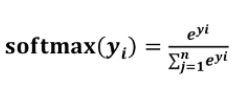softmax 函数应用：在 n 分类中，模型会有 n 个输出，即 y1，y2 ... n, 其中yi表示第 i 中情况出现的可能性大小。将 n 个输出经过 softmax 函数，可得到符合概率分布的分类结果

在 Tensorflow 中，一般让模型的输出经过 softmax 函数，以获得输出分类的概率分布再与标准答案对比，求出交叉熵，得到损失函数，用如下函数实现：

ce = tf.nn.sparse_softmax_cross_entropy_with_logits(logits = y, labels = tf.argmax(y_, 1))
cem = tf.reduce_mean(ce)

具体代码：

#预测酸奶日销量 y，x1 和 x2 是影响日销量的两个因素。
#应提前采集的数据有：一段时间内，每日的 x1 因素、x2 因素和销量 y_。采集的数据尽量多。
#在本例中用销量预测产量，最优的产量应该等于销量。由于目前没有数据集，所以拟造了一套数
#据集。利用 函数随机生成 x1、 x2，制造标准答案 y_ = = x1 + x2，为了更真实，求和后
#还加了正负 0.05 的随机噪声。
#我们把这套自制的数据集喂入神经网络，构建一个一层的神经网络，拟合预测酸奶日销量的函数

#交叉熵(Cross Entropy)：表示两个概率分布之间的距离，交叉熵越大，两个概率分布距离越远，两概率分布越相异；
# 交叉熵越小，两个概率分布 距离 越近 ，两个概率分布越相似
import tensorflow as tf
import numpy as np
BATCH_SIZE = 8
SEED = 23455

#基于seed产生随机数
rdm = np.random.RandomState(SEED)
#随机数返回32行2列的矩阵  表示32组   体积和重量   作为输入数据集
X = rdm.rand(32, 2)
#作为输入数据集的标签（正确答案）
Y_ = [[x1+x2+(rdm.rand()/10.0-0.05)] for(x1, x2) in X]

#定义神经网络的输入、参数和输出，定义前向传播过程
x = tf.placeholder(tf.float32, shape=(None, 2))
w1 = tf.Variable(tf.random_normal([2, 1], stddev=1, seed=1))
y_ = tf.placeholder(tf.float32, shape=(None, 1))    #正确答案，标签
y = tf.matmul(x, w1)    #N*1，预测答案

#定义损失函数以及反向传播方法
#损失函数为交叉熵，反向传播方法为梯度下降
#在Tensorflow中，一般让模型的过输出经过sofemax函数，以获得输出分类的概率分布
# 再与标准答案对比，求出交叉熵，得到损失函数
ce = tf.nn.sparse_softmax_cross_entropy_with_logits(logits=y, labels=tf.argmax(y_, 1))
cem = tf.reduce_mean(ce)

#生成会话session，训练STEP轮
with tf.Session() as sess:
#初始化参数
init_op = tf.global_variables_initializer()
sess.run(init_op)

#训练模型，最关键步骤
STEPS = 20000   #迭代次数
for i in range(STEPS):
start = (i*BATCH_SIZE) % 32
end = start + BATCH_SIZE
sess.run(train_step, feed_dict={x: X[start:end], y_: Y_[start:end]})     #喂数据，不断更新参数
if i % 500 == 0:    #每迭代500次输出一次结果  损失函数loss在所有训练集上的损失值
print("After %d training steps, w1 is: " % i)
print(sess.run(w1))
print("\n")
print("Final w1 is: ")  #打印最终参数w1
print(sess.run(w1))

实现结果（部分截取）：

After 18500 training steps, w1 is:
[[-0.8113182]
[ 1.4845988]]

After 19000 training steps, w1 is:
[[-0.8113182]
[ 1.4845988]]

After 19500 training steps, w1 is:
[[-0.8113182]
[ 1.4845988]]

Final w1 is:
[[-0.8113182]
[ 1.4845988]]


展开全文• 在线性回归中用到的最多的是MSE(最小二乘损失函数)，这个比较好理解，就是预测值和目标值的欧式距离。 而交叉熵是一个信息论的概念，交叉熵能够衡量同一个随机变量中的两个不同概率分布的差异程度，在机器学习中就...
文章目录起因原因最根本的原因有2个原因1交叉熵损失函数原理原因2另一个常说的原因用交叉熵损失函数后还会有梯度消失的问题吗？参考来源链接
起因
因为最开始听一个老师讲的时候把这个问题将偏了，或者说没有说明根本原因，所以现在这个问题把我搞蒙了。
原因
最根本的原因有2个
原因1
在线性回归中用到的最多的是MSE(最小二乘损失函数)，这个比较好理解，就是预测值和目标值的欧式距离。
而交叉熵是一个信息论的概念，交叉熵能够衡量同一个随机变量中的两个不同概率分布的差异程度，在机器学习中就表示为真实概率分布与预测概率分布之间的差异。交叉熵的值越小，模型预测效果就越好。
所以交叉熵本质上是概率问题，表征真实概率分布与预测概率分布差异，和几何上的欧氏距离无关，在线性回归中才有欧氏距离的说法，在分类问题中label的值大小在欧氏空间中是没有意义的。所以分类问题不能用mse作为损失函数。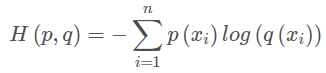交叉熵损失函数原理
参考链接点这里。
以概率分布的角度解释如下:参考链接。
原因2
分类问题是逻辑回归，必须有激活函数这个非线性单元在，比如sigmoid（也可以是其他非线性激活函数），而如果还用mse做损失函数的话：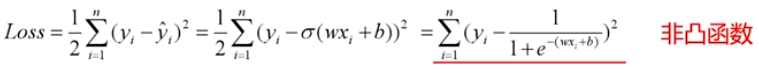mse已经是非凸函数了，有多个极值点，所以不适用做损失函数了。
另一个常说的原因
mse作为损失函数，求导的时候都会有对激活函数的求导连乘运算，对于sigmoid、tanh，有很大区域导数为0的。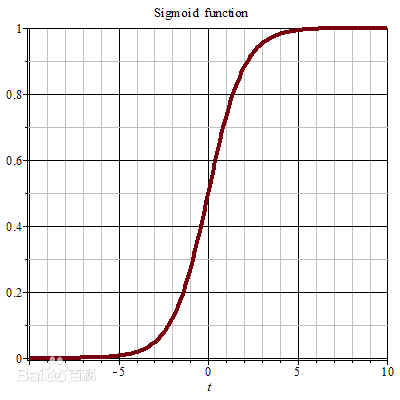该激活函数的输入很可能直接就在平坦区域，那么导数就几乎是0，梯度就几乎不会被反向传递，梯度直接消失了。所以mse做损失函数的时候最后一层不能用sigmoid做激活函数，其他层可以用sigmoid做激活函数。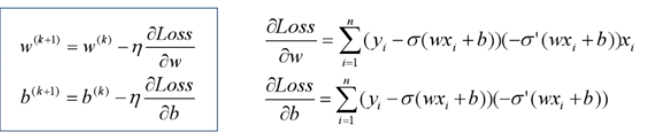当然，用其他损失函数只能保证在第一步不会直接死掉，反向传播如果激活函数和归一化做得不好，同样会梯度消失。所以从梯度这个原因说mse不好不是很正确。
用交叉熵损失函数后还会有梯度消失的问题吗？
梯度消失问题存在2个地方：
1.损失函数对权值w求导，这是误差反向传播的第一步，mse的损失函数会在损失函数求导这一个层面上就导致梯度消失；所以使用交叉熵损失函数。
2.误差反向传播时，链式求导也会使得梯度消失。使用交叉熵损失函数也不能避免反向传播带来的梯度消失，此时规避梯度消失的方法：

ReLU等激活函数；
输入归一化、每层归一化；
网络结构上调整，比如LSTM、GRU等。
深度神经网络，不管用什么损失函数，隐含层的激活函数如果用sigmoid，肯定会梯度消失，训练无效。如果是浅层神经网络，影响可能不是很大，和神经网络的输入有关，如果输入经过了归一化，结果靠谱，如果没有归一化，梯度也直接消失，训练肯定失败。

损失函数和激活函数决定的是模型会不会收敛，也影响训练速度；优化器决定的是模型能不能跳出局部极小值、跳出鞍点、能不能快速下降这些问题的。
参考来源链接
为什么要用交叉熵损失函数
交叉熵损失函数原理详解


展开全文• 第四节最大似然推导mse损失函数（深度解析最小二乘来源)（2）        上一节我们说了极大似然的思想以及似然函数的意义，了解了要使模型最好的参数值就要使似然函数最大，同时损失函数（最小...最小二乘
• 第三节最大似然推导mse损失函数（深度解析最小二乘来源）        在第二节中，我们介绍了高斯分布的来源，以及其概率密度函数对应的参数的解释。本节的话，我们结合高斯分布从数学原理部分解释...最小二乘
• 第三节最大似然推导mse损失函数（深度解析最小二乘来源） 在第二节中，我们介绍了高斯分布的来源，以及其概率密度函数对应的参数的解释。本节的话，我们结合高斯分布从数学原理部分解释为什么损失函数是最小二乘。...
• 第四节 最大似然推导mse损失函数（深度解析最小二乘来源）（2） 上一节我们说了极大似然的思想以及似然函数的意义，了解了要使模型最好的参数值就要使似然函数最大，同时损失函数（最小二乘）最小，留下了一个问题...
• 上面虽然使用的是Sigmoid函数，其实分类问题使用的是softmax函数，softmax可以看做sigmoid在对分类问题上的推广。 import matplotlib.pyplot as plt import numpy as np A = np.linspace(0, 1, 100) plt.plot(A,...
• 今天来讲下之前发的一篇极其简单的搭建网络的博客里的一些细节（前文传送门） 之前的那个文章中，用...loss_func = nn.MSELoss() 那么其中的额SGD和MSE究竟是什么呢？ 一、SGD方法 我们要想训练我们的神经网络，就...神经网络 python pytorch
• 我有一个CNN，我用它来检测面部关键点。我可以画出地面的真实...我读到MSE是回归问题的一个很好的选择，我把损失函数改为MSE，现在我得到的所有训练都是nan作为我的损失。我已经系统地改变了参数，例如lr从0.1-0.00...
• ## MSELoss损失函数

千次阅读 2020-07-10 11:40:08
MSELoss损失函数中文名字就是：均方损失函数，公式如下所示： 这里 loss, x, y 的维度是一样的，可以是向量或者矩阵，i 是下标。 很多的 loss 函数都有 size_average 和 reduce 两个布尔类型的参数。因为一般...
• 损失函数损失函数、代价函数、目标函数）、MSE、0-1损失函数、绝对误差损失函数、分位数损失函数、Huber损失函数、感知损失函数、Hinge损失函数、指数损失函数、对数损失函数 目录 损失函数损失函数、代价函数...机器学习 深度学习 逻辑回归 神经网络 数据挖掘
• MSELoss损失函数中文名字就是：均方损失函数，公式如下所示： 这里 loss, x, y 的维度是一样的，可以是向量或者矩阵，i 是下标。很多的 loss 函数都有 size_average 和 reduce 两个布尔类型的参数。因为一般损失函数...
• ## pytorch的nn.MSELoss损失函数

万次阅读 多人点赞 2018-07-13 13:25:49
1、均方损失函数： loss(xi,yi)=(xi−yi)2loss(xi,yi)=(xi−yi)2\text{loss}(\mathbf{x}_i, \mathbf{y}_i) = (\mathbf{x}_i - \mathbf{y}_i)^2 这里 loss, x, y 的维度是一样的，可以是向量或者矩阵，i 是下标。 ...
• MSELoss损失函数中文名字就是：均方损失函数，公式如下所示： 这里 loss, x, y 的维度是一样的，可以是向量或者矩阵，i 是下标。 很多的 loss 函数都有 size_average 和 reduce 两个布尔类型的参数。因为一般...
• 总结对比MSE损失函数，MAE损失函数以及Smooth L1_loss损失函数的优缺点 1、常见的MSE、MAE损失函数 1.1、均方误差MSE 均方误差（Mean Square Error,MSE）是回归损失函数中最常用的误差，它是预测值f(x)与目标值y之间...
• 1、均方差损失函数 loss, x, y 可以是向量或者矩阵，i 是下标。 很多的loss函数都有size_average和reduce两个布尔类型的参数。因为一般损失函数都是直接计算 batch 的数据，因此返回的loss 结果都是维度为 ......